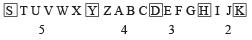Courses

# Olympiad Test : Alphabet Test - 1

## 20 Questions MCQ Test Maths Olympiad Class 6 | Olympiad Test : Alphabet Test - 1

Description
This mock test of Olympiad Test : Alphabet Test - 1 for Class 6 helps you for every Class 6 entrance exam. This contains 20 Multiple Choice Questions for Class 6 Olympiad Test : Alphabet Test - 1 (mcq) to study with solutions a complete question bank. The solved questions answers in this Olympiad Test : Alphabet Test - 1 quiz give you a good mix of easy questions and tough questions. Class 6 students definitely take this Olympiad Test : Alphabet Test - 1 exercise for a better result in the exam. You can find other Olympiad Test : Alphabet Test - 1 extra questions, long questions & short questions for Class 6 on EduRev as well by searching above.
QUESTION: 1

### ​Arrange the given words in alphabetical order and choose the one that comes first.

Solution:

Groan, Grotesque, Group, Guarantee.

QUESTION: 2

### ​​Arrange the given words in alphabetical order and choose the one that comes first.

Solution:

Nature, Nautical, Naval, Necessary.

QUESTION: 3

### ​​Arrange the given words in alphabetical order and choose the one that comes first.

Solution:

Foetus, Foliage, Foment, Forceps.

QUESTION: 4

​​​Arrange the given words in alphabetical order and choose the one that comes first.

Solution:

Deuce, Devise, Dew, Dexterity.

QUESTION: 5

​​​Arrange the given words in alphabetical order and choose the one that comes first.

Solution:

Qualify, Quarrel, Quarry, Quarter.

QUESTION: 6

​​​​Arrange the given words in alphabetical order and choose the one that comes first.

Solution:

Probate, Probe, Proceed, Proclaim.

QUESTION: 7

​Arrange the given words in the alphabetical order and choose the one that comes last.

Solution:

Fault, Finger, Floor, Forget.

QUESTION: 8

​​Arrange the given words in the alphabetical order and choose the one that comes last.

Solution:

Evolution, Extra, Extraction, Extreme.

QUESTION: 9

​​Arrange the given words in the alphabetical order and choose the one that comes last.​

Solution:

Translate, Transmit, Transport, Transist.

QUESTION: 10

​​​Arrange the given words in the alphabetical order and choose the one that comes last.​

Solution:

Repeat, Roman, Romance, Rose.

QUESTION: 11

​​​Arrange the given words in the alphabetical order and choose the one that comes last.​

Solution:

Diagonal, Dialogue, Different, Distance.

QUESTION: 12

​​​​Arrange the given words in the alphabetical order and choose the one that comes last.​

Solution:

Transition, Transmit, Temperature, Temple.

QUESTION: 13

​​​​Arrange the given words in the alphabetical order and choose the one that comes last.​

Solution:

Waiting, Warring, Watching, Waving.

QUESTION: 14

In each of the following questions find out which of the letter series follows the given rule.
Q . Number of letters skipped in between adjacent letters in the series is odd.

Solution: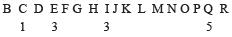1, 3, 5 are all odd numbers.

QUESTION: 15

In each of the following questions find out which of the letter series follows the given rule.
Q. Number of letters skipped in between adjacent letters in the series is in the order of 2, 5, 7, 10.

Solution: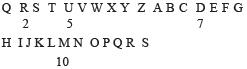QUESTION: 16

In each of the following questions find out which of the letter series follows the given rule.
Q. ​Number of letters skipped in between adjacent letters in the series decreases by three.

Solution: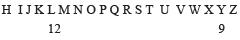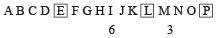QUESTION: 17

In each of the following questions find out which of the letter series follows the given rule.
Q. ​Number of letters skipped in between adjacent letters in the series decreases by one each time.

Solution: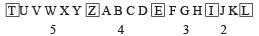QUESTION: 18

​In each of the following questions find out which of the letter series follows the given rule.
Q. Number of letters skipped in between adjacent letters in the series doubles every time.

Solution: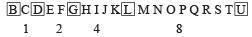QUESTION: 19

​In each of the following questions find out which of the letter series follows the given rule.
Q. Number of letters skipped in between adjacent letters of the series starting from behind increases by one.

Solution: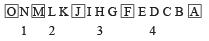QUESTION: 20

​In each of the following questions find out which of the letter series follows the given rule.
Q. Number of letters skipped in between adjacent letters decrease in order.

Solution: